# Bringing Sequential Testing to Experiments with Longitudinal Data (Part 1): The Peeking Problem 2.0July 18, 2023

# Spotify’s approach to challenges in sequential testing with longitudinal data

At Spotify, we’re constantly improving our data infrastructure, which means we can get feedback on experiments earlier and earlier. To allow for early feedback in a risk-managed manner, we use sequential tests to monitor regressions in the experiments. However, when moving toward smaller and smaller time windows, we’re faced with multiple measurements per unit in our analysis, which is known as longitudinal data. This, in turn, introduces new challenges for designing sequential tests. While papers like Guo and Deng (2015) and Ham et al. (2023) discuss this type of data and its use in experimentation, the challenges we present haven’t to the best of our knowledge been fully addressed in the online experimentation community. Our approach to addressing these challenges is presented in two parts:

Part 1: The peeking problem 2.0 and other challenges that occur when applying standard sequential tests in the presence of multiple observations per unit

Part 2: General advice for performing sequential tests in the presence of multiple observations per unit, and how Spotify uses group sequential tests (GSTs) for a large class of estimators

# The Peeking Problem 2.0

Continuous monitoring of A/B tests is an integral part of online experimentation. Sequential testing, although introduced in statistics and used in clinical trials for over 70 years (Wald, 1945), was not widely adopted in the online experimentation community until it was popularized by papers like Johari et al. (2017) and others. The standard “peeking problem” occurs when people running an experiment conduct statistical analysis on the sample before all participants’ results have been observed, which leads to an inflation of the false positive risk (Johari et al. 2017) and violates the statistical assumptions of the test.

Sequential testing offers a solution to the peeking problem as it enables peeking during the experiment without inflating the false positive rate (FPR). There is now a rich literature on sequential testing. For a comprehensive overview and comparison of different sequential testing frameworks, see our previous blog post

However, we’ve observed a new problem, which we call the “peeking problem 2.0” — a problem that can substantially inflate false positive rates despite the use of sequential tests. This problem is relevant for data where each participant is measured at multiple points in time during the experiment, and it can occur when a participant’s results are peeked at before all the measurements of that participant have been collected (“within-unit peeking”).

To illustrate the challenges that arise with longitudinal data, we will study the following two common types of metrics:

1. Cohort-based metrics. Where units are measured over the same, fixed time window after being exposed to the experiment. These metrics do not suffer from the peeking problem 2.0. However, these metrics force the experimenter to either wait longer for results or use less of the available data.
2. Open-ended metrics. Where metrics are based on all available data per unit. These metrics are highly likely to be affected by the peeking problem 2.0 because standard sequential tests are typically invalid. However, they are appealing because they always utilize all available data. Open-ended metrics are common in practice and supported by several online experimentation vendors.

To enable a detailed discussion, we first discuss the need to be precise with the underlying statistical goal. When multiple measurements are available per unit, it becomes more important to be clear on which type of treatment effect we’re trying to learn about. Then, we give an overview of cohort-based metrics and open-ended metrics, discuss their trade-offs, and explain why an open-ended metric poses special challenges for sequential statistical analysis. By conducting a small Monte Carlo simulation study, we show that using standard sequential tests for open-ended metrics can lead to substantially inflated false positive rates.

## Longitudinal data and when to measure outcomes

Although the development of infrastructure for collecting data has made huge strides forward, this has not been reflected in the literature on sequential testing. This development has made it possible to measure units and aggregate results more frequently during the experiment. Interestingly, even though the literature on sequential testing is heavily focused on the ability to continuously monitor outcomes during experiments, there has been little discussion about how to incorporate more granular measurements in sequential tests in a valid manner. For example, to understand how much audio content a Spotify user consumes — and assuming a user needs time to listen — measuring the difference in music consumption within the first five seconds wouldn’t yield distinguishable results. The user would not have had the time to exercise their potentially changed behavior under the treatment. In other words, without being able to incorporate repeated measurements per unit in the experiment analysis, small time-window measurements inevitably create a conflict between early detection and utilizing all the data available for a unit. So should we measure units (e.g., users or customers) for a very short time after they enter the experiment so that the test can be performed early, or should the unit be measured for a longer time window so that we learn more about the unit’s response? The only way to have the cake and eat it too is to consider repeated measurements per unit in the sequential analysis.

Figure 1 displays different data collection patterns. Each box represents one observation made available for analysis where the corresponding box ends. The Short and Long panels record only one observation per unit, where the difference is the time window in which the observation is calculated. This aggregation into only one observation implies a trade-off between getting a measurement fast versus observing the unit for long enough. In the repeated panel, all measurements per unit are used as observations, and there is no trade-off between the two. However, statistical analysis of panels of repeated measurements per unit (i.e., longitudinal data) is more complex.

### Separating the concepts makes it easier to solve the problem

It’s easy to confuse metrics on the one hand, and estimands and statistical tests regarding them on the other. However, to derive valid and efficient sequential tests in more general data settings, we find that it is crucial to separate between two important questions:

1. What behavior or aspect will be measured per unit, and how often and over what time windows will this be measured?
2. What treatment effect, estimator, and statistical tests will be used to evaluate if the treatment is performing better than the control?

Many online experimenters are so used to working with average treatment effects and standard difference-in-means estimators that the measurements, the metric, the estimand, the estimator, and the statistical test are all conflated. To make this concrete, consider the following example: say that we are testing a change for which the alternative hypothesis is that music consumption will increase. The outcome is measured using the metric “average minutes played over a week”, which is a common metric at Spotify. This metric is based on a single observation per user, their total minutes played over a week, which, in turn, is based on more granular measurements. These more granular measurements are typically hourly or daily.

It’s standard practice to compare the difference-in-means between the treatment and control group. This is where the risk of conflation of concepts begins to increase. The difference-in-means estimator is often an unbiased estimator of the average treatment effect on the metric in question. However, there are many unbiased estimators for the average treatment effect on any given metric. A common alternative to the difference-in-means estimator is the covariate-adjusted regression estimator — of which CUPED (Deng et al. 2013) is a special case. For many estimators, there are also several possible statistical inference methods. For both the difference-in-means estimator and the covariate-adjusted estimator, there are many ways to conduct inference, e.g., asymptotic normal theory–based t- and z-tests, randomization inference, or bootstrap. There are, of course, many other interesting estimands for any given metric, for example, the difference-in-median. Additionally, it might be preferable for the same set of measurements to use group-level aggregated metrics — sometimes called ratio metrics and click-through rate metrics — where no within-unit aggregation across measurements is performed.

When extending sequential testing to longitudinal data settings, it becomes increasingly important to be clear about the separation of measurements, metrics, estimands, estimators, and inference.

### The data and statistical goal of an online experiment

In the most basic of experiments, the goal is to assess whether a treatment is “better” than a control. By randomly distributing units — such as users — to either treatment or control, the only difference between units in the two groups is which experience they have been randomly allocated to. The randomization allows us to causally claim that any difference that we see between the two groups in metrics is because of the treatment. To more clearly see how we formulate the difference, or in what sense treatment is better than control, we need to introduce some notation.denote the observed potential outcome for unit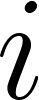at time. This could be, for example, music consumption during a day or number of app crashes during an hour at theth measurement after the unit was exposed to either treatment () or control (). During the data collection of an experiment, the sequence of observed measurements will typically be a mix of repeated measurements of users that have already been observed at least once, and the first measurement of new users.

To illustrate some details and concepts, consider the following example of a small sample. We assume data is delivered in batches. For example, this could be daily measurements being delivered after each day has passed. In this example, the observed samples of the three first batches are shown in Figure 2.Figure 2: Example data from six users. Columns are days and rows are users. Yᵢ,ⱼ(d) denotes an observation for user i on day j with treatment d. Users 1 (treated) and 2 (control) are first observed on day 1 and subsequently once every day. Users 3 and 4 are first observed on day 2, and 5 and 6 on day 3.

Here, when the third batch has come in, the first and second units are observed three times, whereas the third and fourth units are only observed twice, and the fifth and sixth units are observed only once. When samples of measurements like this are available, there are many options available for testing the difference between treatment and control.

## Cohort-based and open-ended metrics are two common ways of aggregating data

The terms “cohort-based metrics” and “open-ended metrics” are sometimes casually used to describe two distinct types of metrics. These are not the only types of metrics and are arguably in themselves causing conflation of statistical concepts, as discussed above. However, they serve as an excellent tool for illustrating the trade-offs and challenges of working with longitudinal data in a sequential testing setting, and we define them next.

### Cohort-based metrics

A cohort-based metric is centered around the concept of time since exposure (TSE). For example, at Spotify, we rely on days since exposure and define metrics such as “average minutes played during the first seven days of exposure”. For this metric, only users with at least seven days of exposure have a value for this measurement. This means that the data from users with six or fewer days of exposure at a given intermittent analysis is excluded from that analysis. All measurements collected for users after their seventh day of exposure are also discarded from the calculation of the metric. Moreover, the first analysis cannot be done until seven days into the experiment, and at that point it will only be the units that came into the experiment the first day that are included in the analysis.

Let’s make this a bit more precise. A cohort-based metric is defined for fixed windows of measurements in relation to the time since exposure. For example, Figure 3 displays the available data for a given user, where the boxes represent daily data points.Figure 3: Hypothetical data for a given user during the first seven days of exposure to an experiment.

An example of a cohort-based metric could be the average across users of the total during days 3 and 4. In this case, the user in the example would contribute with the measurement 12+16=28 on day 4, and would not have been measured prior to day 4. Later data points are not used, and the user’s measurement, 28, remains fixed. The metric could use other forms of aggregations — the primary feature of the cohort-based metric is that each unit comes into the analysis with one observation, and that observation is fixed between analyses.

Let’s now return to the earlier small example dataset with three batches. Define the metric as the average over the first two measurements after exposure. With the given observed measurements, the cohort-based metric would not be defined for any units when only the first batch is observed. At the second batch, the sample in terms of the metric would be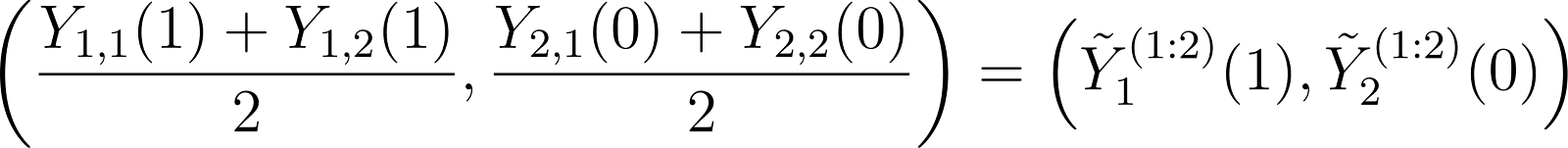where measurements are discarded. When the third batch comes in, we have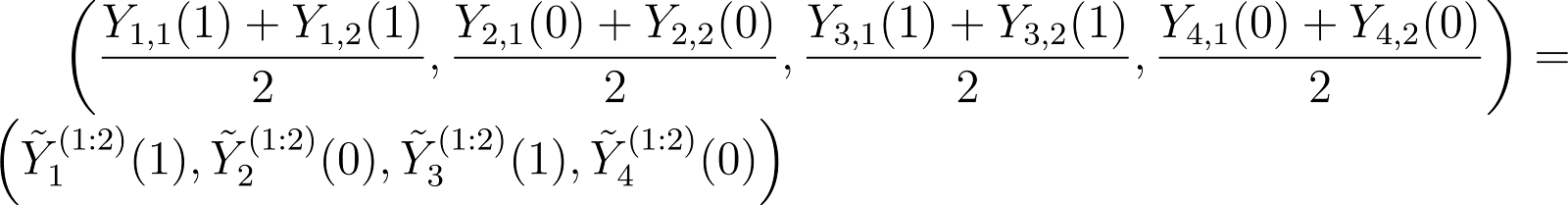Let’s now study the difference-in-means estimator on this metric. For the first batch, again, there are no units with observed metrics. When the second batch comes in, the estimator will be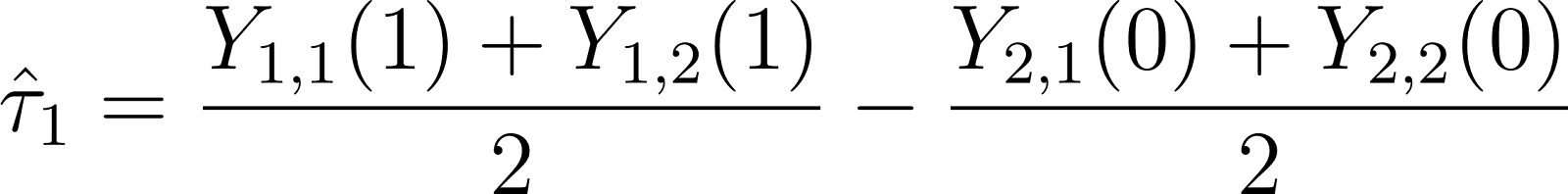At the third batch, we have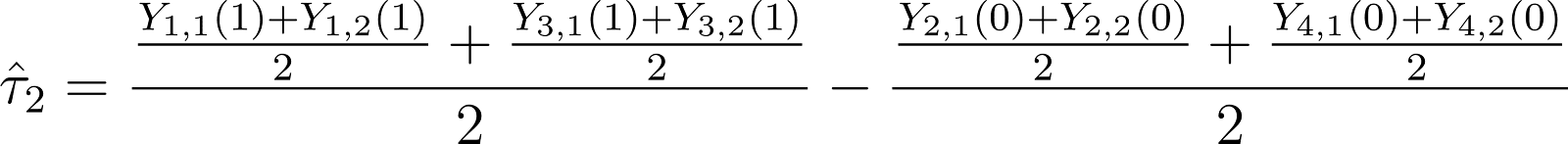For the comparison to open-ended metrics below, note that, for any given cohort-based metric, the estimator at any given intermittent analysis yields an unbiased estimator of the same estimand. Let’s assume for simplicity thatand, thus the average treatment effect follows the pattern. That is, treatment effects are equal across units, but different over time. The expected value of the estimator at the first possible analysis is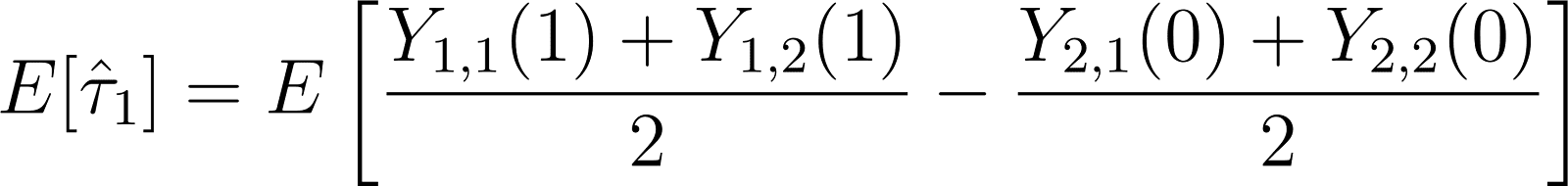For the second analysis, we have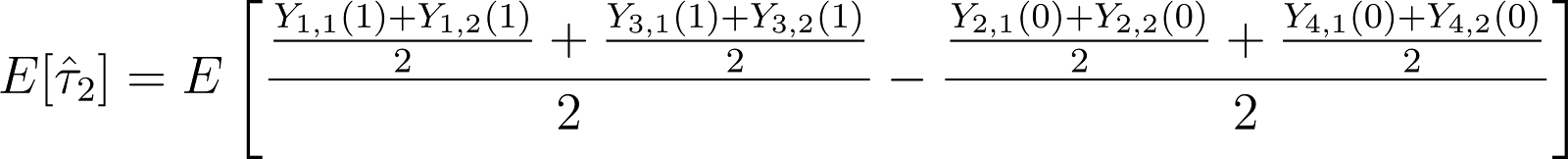That is, at any intermittent analysis where the metric is defined for some units, the estimator is an unbiased estimator of the average treatment effect across the two first measurements after exposure.

#### The enforced trade-off between early detection and data utilization

For cohort-based metrics, the experimenter needs to choose between early detection and data utilization. That is, if the cohort-based metric is defined over many measurements, units will not have any metric values until they have at least that many measurements observed. At the same time, if the cohort-based metric is defined over only, say, the first measurements, all measurements observed after that will be discarded for each unit. It is possible to define several cohort-based metrics, one for each days-since-exposure offset, but then the analysis will need to account for multiple testing with reduced sensitivity as a consequence.

### Open-ended metrics

An alternative to a cohort-based metric is an open-ended metric. With an open-ended metric, all available data is used at each analysis time. In practice this can mean different things, for example, the average treatment effect on the within-unit aggregate, or the median treatment effect on the average daily consumption across units and days, etc. In most cases, an “open-ended” metric is very similar to a cohort-based metric. The main difference is that, regardless of aggregation function, the metric is defined per unit from when the first measurement is observed per unit, and all measurements after that are included (hence “open-ended”).

Consider again the hypothetical data from Figure 3. With an open-ended metric based on within-user averages, this user would contribute the measurement 15/1 on the first day; on the second day, their contribution would be (15+10)/2; on the third day, it would be (15+10+12)/3; and so on. With an open-ended metric, units are measured as soon as possible, and all available data is used.

Open-ended metrics are not uncommon in practice, and they’re supported by several commercial online experimentation vendors. Open-ended metrics are appealing because they can leverage all of the available data at any given point in time and appear similar to metrics used in dashboards and reports. However, as we will see in the next section, that similarity hinges on strong assumptions that are unlikely to be met in practice.

#### The moving target of open-ended metrics makes (sequential) statistical inference challenging

An important difference between open-ended metrics and cohort-based metrics is that, for open-ended metrics, the measurement for a given unit changes between intermittent analyses. This means that any estimator based on a metric on top of these measurements will vary between intermittent analyses for two reasons: both due to new units entering the experiment and because new measurements become available for already observed units, thereby updating each unit’s “measurement”. This poses a major challenge for deriving valid sequential tests.

Furthermore, the difference-in-means estimator on an open-ended metric is difficult to interpret because the population parameter for which it is an unbiased estimator is changing between intermittent analyses. We again return to the small example dataset with three batches. The difference-in-means estimator on the open-ended metric with the within-unit mean as the aggregation function would be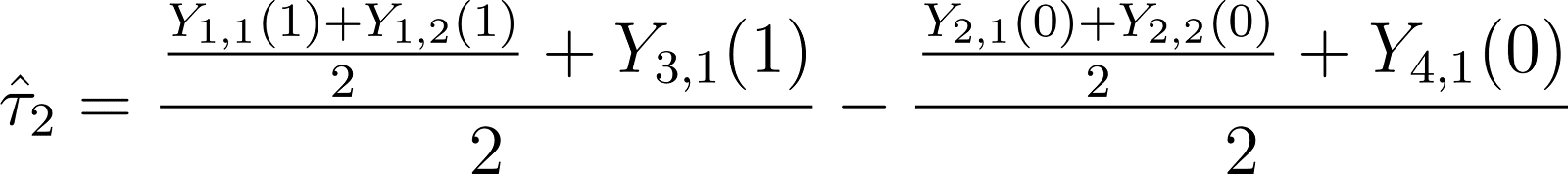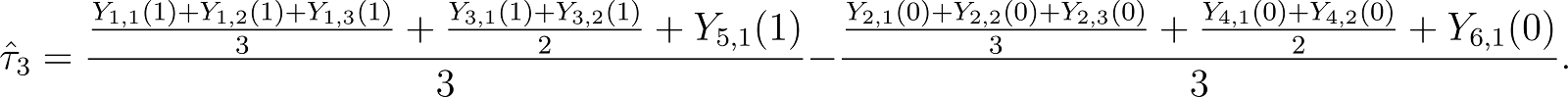Using the same mean and treatment structure as for the cohort-based example, for the open-ended metric, the expected value of the estimator at the first analysis isAt the second analysis, the expected value of the estimator is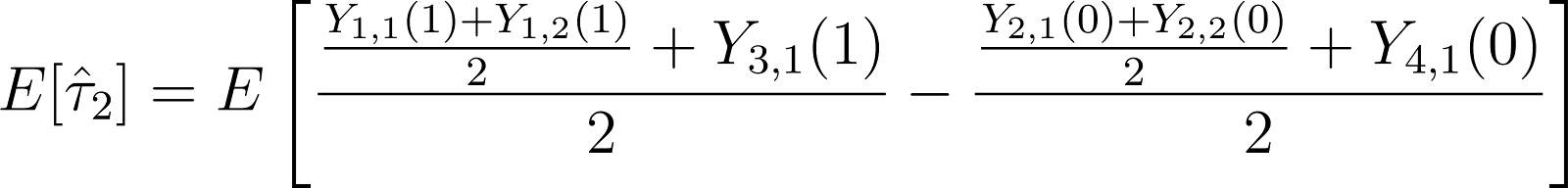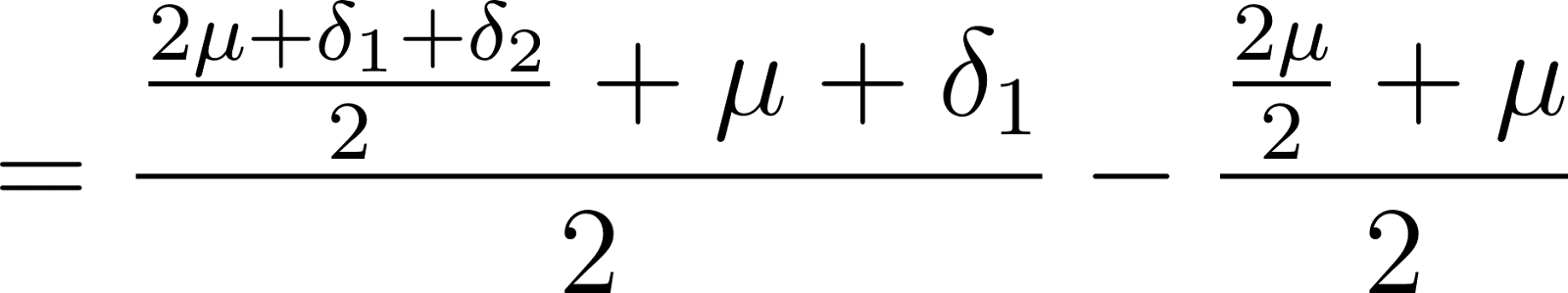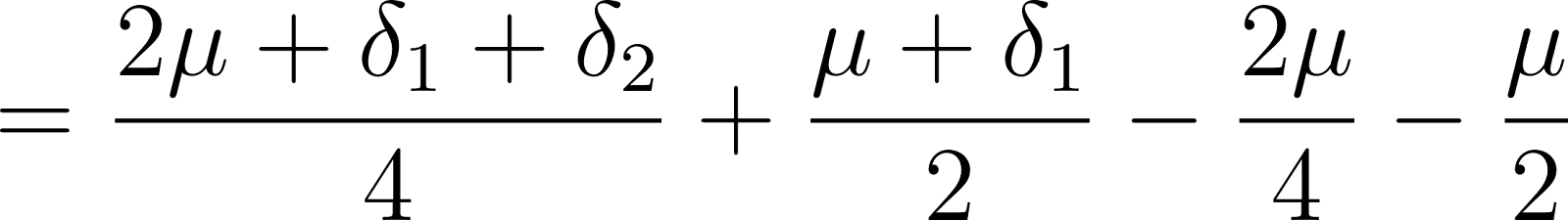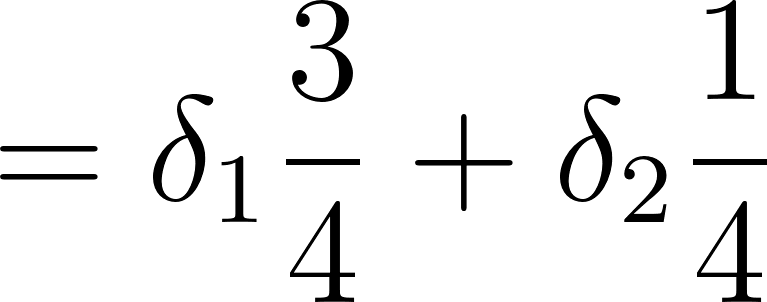And, finally,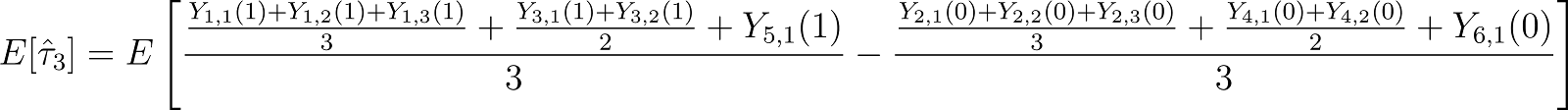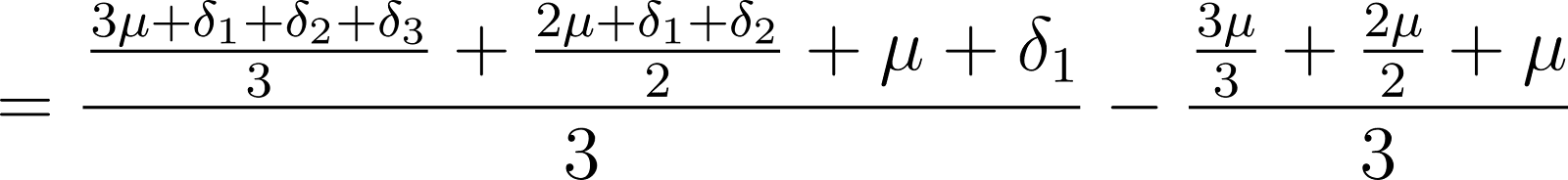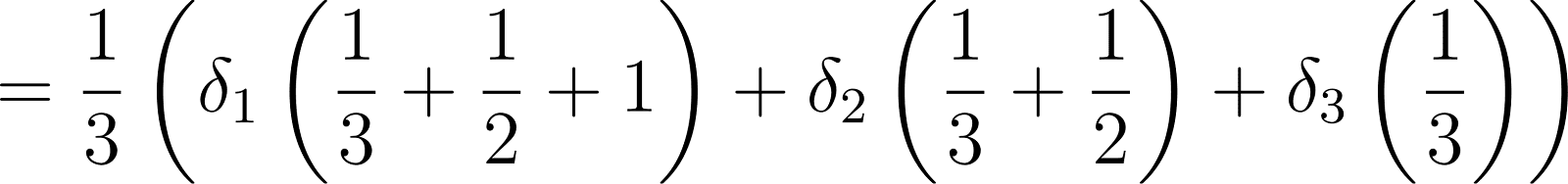As we can see, the implied estimand changes between analyses for open-ended metrics, which stands in contrast to cohort-based metrics. Due to the within-unit means changing between intermittent analyses, the estimand becomes an increasingly more complicated weighted sum of the three first-time treatment effects. In practice, the weights will be a function of the intake distribution, which in most cases is an arbitrary way to select the weights — especially if ramp-up or other gradual schemes for increasing the reach of the experiment are used.

One challenge with the changing estimand is that standard sequential tests are typically derived for a fixed parameter of interest. Since open-ended metrics imply an estimand that may change from analysis to analysis, more care needs to be taken when deriving sequential tests. Using standard sequential tests can lead to severely inflated FPRs.

### A small Monte Carlo study

To illustrate the possible FPR inflation from using the open-ended metric approach in the presence of longitudinal data, we conduct a small simulation study.

As a benchmark, we include treating the measurements within and across units as all independent and identically distributed. This is a naive approach, which will be incorrect by construction if the within-unit correlation is non-zero. We perform 1,000 replications per setting.

We vary two parameters in the simulation design:

• K: The max number of measurements per unit is set to K=1, 5, 10, 20.
• AR(1): The coefficient of the within-unit autoregressive correlation structure is set to 0 and 0.5.

Data is generated as follows. For each simulation design, we generate N=1,000*K multivariate normal observations with a within-unit correlation structure given by an AR(1) process with the given parameter value. We let N increase with K to ensure enough units are available per measurement and analysis. In the simulation, units come into the experiment uniformly over the first K time periods, which means that all units will have all K measurements observed at the 2K-1th time period, which is, therefore, the number of intermittent analyses used per replication. That is, the units that entered the experiment at time period K will need K-1 additional days to have all K measurements observed. The tests are performed with alpha set to 0.05.

In the simulation, we compare three methods:

1. IID: Standard GST, treating each measurement within and between units as IID. The expected sample size is set to N*K.
2. Open-ended Metric: Standard GST, applied to the open metric as defined above. The experiment ends when the last units have their first measurements observed. This implies that not every unit reaches their max number of measurements.
3. Open-ended Metric (oversampled): Same as Open-ended Metric, but the experiment is continued until all units have all K measurements observed.

We include both “Open-ended Metrics” and “Open-ended Metrics (oversampled)” to illustrate that there are multiple mechanisms at play that drive the false positive inflation. When implementing these tests, it immediately becomes clear how awkward it is to use standard sequential tests for open-ended metrics. For a standard GST, the full sample (in terms of number of units) is collected as soon as the last unit has gotten its first measurement observed. This means that all alpha is spent already at the Kth analysis, after which the test statistic still updates due to new measurements coming in implying additional K-1 analyses. This inevitably leads to an inflated FPR. This is in addition to the inflated FPR that occurs due to the unmodeled covariance within units.

#### Results

Figure 4 displays the results. The x axis is the maximum number of repeated measurements per unit. The y axis is the empirical false positive rate. The colors correspond to three methods: 1) IID treating all measurements across units and time points as IID; 2) OM using a within-unit mean open-ended metric stopping intake once all units have at least one measurement; and 3) OM (oversampled), which is the same as OM, but data collection continues until all units have all measurements. The bars indicate 95% normal-approximation confidence intervals for the true false positive rate. The two panels display the results for the three methods under each correlation structure, respectively. For both settings, when we only have one measurement per person, the sample is simply the N IID observation being analyzed once. That is, the GST reduces to fixed-horizon tests, and all variants should have nominal alpha levels.

In the IID case, when the within-unit measurements are independent (AR(1)=0) the FPR is centered on alpha across numbers of measurements. Using the IID method, as soon as the within-unit correlation over time is nonzero, the false positive inflation increases accordingly.

For the two open-ended metric methods, the false positive rate inflates as the number of measurements per unit increases. As expected, the FPR is higher in the oversampled case, but it only explains a small portion of the FPR inflation. Here, the pattern is somewhat opposite to the IID case, in that the higher the within-unit autocorrelation, the less inflated the FPR. This is expected, as when the within-correlation increases, the amount of new information in the within-unit mean from a new observation goes down. If the within-unit correlation approaches 1, the open-ended metric would not vary within units over time, and thus the peeking problem 2.0 wouldn’t occur.

In summary, without taking the within-unit covariance into account, there is a high risk that we will end up inflating the FPR, despite using sequential tests. While we focused on GSTs in the simulation here, the same problem can occur in other families of sequential tests that don’t account for the within-unit covariance. Due to the peeking problem 2.0, we cannot expect guarantees on false positive rates to hold unless we adjust the sequential test accordingly.

## Conclusion

More frequent measurements enable faster feedback in online experiments. However, more frequent measurements imply repeated measurements per unit, a type of data known as  longitudinal data. In terms of sequential testing, moving from a single observation per unit to repeated observations per unit introduces new challenges. In this post, we have shown that without addressing the potentially more complex covariance structure of the estimator between intermittent analyses, the false positive rate can be severely inflated. This is caused by the peeking problem 2.0 — peeking on units’ results before all their measurements are observed.

The original peeking problem stems from the fact that we are using a test that assumes the full sample is available at the analysis before the full sample is available. The peeking problem 2.0, on the other hand, stems from the fact that we are using a sequential test that assumes that a unit’s measurement is finalized when entering the analysis when it is not. The mechanisms that cause the issues are different, but the consequences are the same. To handle these challenges and derive proper sequential tests for metrics based on multiple observations per unit, it’s important to be explicit about the estimand and the estimator — not all sequential tests are valid in all settings!

In Part 2 of this blog post series, we give advice on how to perform sequential tests with longitudinal data, including practical considerations and what tests we’re using at Spotify.

## Acknowledgments

The authors thank Lizzie Eardley and Erik Stenberg for their valuable contributions to this post.

Tags: